﻿ Application of Artificial Intelligence to Predict Compressive Strength of Concrete from Mix Design Parameters: A Structural Engineering ApplicationJournal of Civil Engineering Research

p-ISSN: 2163-2316    e-ISSN: 2163-2340

2015;  5(6): 158-161

doi:10.5923/j.jce.20150506.05### Application of Artificial Intelligence to Predict Compressive Strength of Concrete from Mix Design Parameters: A Structural Engineering Application

M. A. Faruqi1, R. Agarwala2, J. Sai1, A. Francisco1

1Department of Civil Engineering, MSC 194, Texas A & M University – Kingsville, Kingsville, TX, USA

2College of Technology and Science, Suite 100 Science and Technology Building, Greenville, NC, USA

Correspondence to: M. A. Faruqi, Department of Civil Engineering, MSC 194, Texas A & M University – Kingsville, Kingsville, TX, USA.
 Email: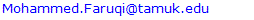Abstract

There are no fixed formulations for mixing concrete constituents to obtain the compressive strength. Concrete mixing is predominantly a qualitative knowledge-based approach subjected to variations. Reliance on such an approach compromises the precision and accuracy of concrete properties, and hence necessitates the development of a reliable mixing formulation. Statistical modeling techniques like Multiple Linear Regression Analysis (MLRA) have been used in the past. However, these methods have failed to accurately predict the compressive strength. This is due to the highly nonlinear relationship between the concrete proportions and its properties. In this paper a Neural Network model for predicting the compressive strength of concrete for different mix-design parameters is developed. A neural network model based on five hidden layers was trained using the results of a series of previously conducted experiments. Each experiment consisted of five parameters and a corresponding compressive strength obtained from 28-days cylinders tests. It was observed that the neural network model performed with satisfactory results in predicting the 28-day compressive strength of concrete.

Keywords: Concrete, Compressive Strength, Neural Networks, Mix-Design Parameters

Cite this paper: M. A. Faruqi, R. Agarwala, J. Sai, A. Francisco, Application of Artificial Intelligence to Predict Compressive Strength of Concrete from Mix Design Parameters: A Structural Engineering Application, Journal of Civil Engineering Research, Vol. 5 No. 6, 2015, pp. 158-161. doi: 10.5923/j.jce.20150506.05.

### 1. Introduction

Concrete is a man-made material obtained by mixing of cement, coarse aggregates, fine aggregates, and water. Admixtures are sometimes added to alter the properties of concrete. It is also known that by controlling some parameters of fresh concrete, such as: aggregates, cement grade, and water/cement ratio, the long-term properties of the concrete can be improved. Compressive strength of concrete is an important property, which is generally obtained after a standard curing of 28 days and testing using a Materials Testing Machine (MTS). Conventional methods of predicting 28-day compressive strength are based on statistical analyses. These analyses have led to the development of many linear and some nonlinear equations to model such a problem inaccurately . This work makes an attempt to utilize artificial neural networks (ANN) to predict the compressive strength. An ANN is a simplified mathematical model or computational tool that tries to simulate the structure for engineering problems. It contains an interconnected group of artificial neurons and processes information using a connectionist approach to computation. In most cases the ANN is an adaptive system that changes its structure based on external or internal informattion that flows through the network during the learning phase and can be used as a prediction tool for cases where the output solution is not available . Modern neural networks are non-linear statistical data modeling tools. They are used to model complex relationships between inputs and outputs or to find patterns in data.
Very few works have addressed the application of neural networks in material modeling. Snell and Roekel  developed an automatic knowledge based on a multilayer feed-forward principle for mix designs. Oh, et al.  used a back-propagation network for concrete mixes. Lai and Serra  developed a neuro-computing model for predicting with sufficient accuracuy the compressive strength of cement conglomerates. Brown et al.  demonstrated the applicability of neural networks for composite material characterization. Ghaboussi et al.  modeled the behavior of concrete under a state of plane stress using monotonic biaxial loading and compressive uniaxial loading with a back propagation neural network. This paper involves mapping the influencing parameters to predict the 28-day compressive strength of concrete using neural networks. Different mapping models with different hidden layers were created and subjected to various training cycles. Finally, a neural network model based on the accuracy of strength validation and Mean Square Error (MSE) was selected and is presented here.

### 2. Basics of Modeling

Neural networks (NN) contain three main sections which are classified as, input layer, hidden (inner) layer, and output layer. Input parameters are presented in the input layer and the solution of the problem is evaluated with the output layer. In between these layers, hidden layer is placed and provides help to the network in the learning process. The number of neurons of the input and output layers are determined in order to represent the characteristic of the existing problem accurately. Hidden layers can be formed with one or more layers and the number of neurons in the hidden layer is determined by the user. In general, NN model applications are performed in two steps. The network is created and trained at the first step and the network is validated with new input variables at the second step. During the training phase, net information is transmitted to output layer with connection weights.
Key advantages of the neural network (NN) are its ability to learn, recognize, generalize, classify and interpret incomplete and noisy inputs and its ability to represent both linear and nonlinear relationships with great accuracy.
This problem is defined as a nonlinear input-output relation between the influencing factors and 28-day compressive strength of concrete. This led us to choose 5-5-1 architecture. This means there are five nodes in the input layer corresponding to the five parameters, five in the hidden layers, and one in the output layer corresponding to 28-day compressive strength. Fig1 shows the architecture. The neurons of neighboring layers are fully connected.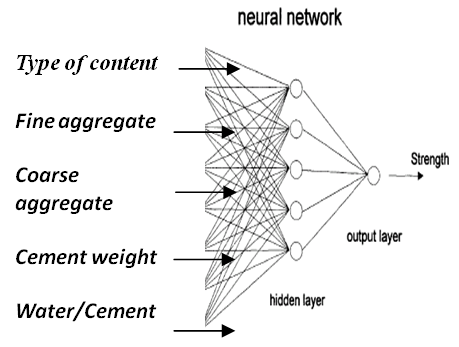Figure 1. The architecture of network
Data from 28 experiments were obtained by means of extensive and detailed laboratory work conducted by the author and his assistant . The experiments were divided into two sets, one for the network learning called learning set, and the other for validating the network called testing set. Each set consisted of input vectors and output vectors. An input vector consisted of five components as shown in Fig 1. Output vector had only one strength component. There were twenty pairs of vectors in the learning set and eight in the testing set.

### 3. Simulation and Discussion of Results

A Feed forward (FF) network model based on the accuracy of strength validation and mean square error (MSE) was developed. This is because FF model has connections and can jump over one or more layers to provide better efficiency in results. Fig. 2 shows the basic chosen neural network model. Table 1 shows the training and the testing results. A training cycle of 1000 epochs up to a maximum limit of 10,000 epochs were chosen based on the quality of the output. In the final neural model, five processing elements were input. These were: content type, fine aggregates, coarse aggregates, cement type, and water to cement ratio. In each of the five hidden layers, a tangential function for processing elements was adopted in the neural model. This is because this function helped in convergence of results at a faster rate. Also, in all of the five hidden layers, the step size was increased by 0.1. However, the momentum was kept at 0.7. This decrease in step size and a constant computational momentum provided accurate results. The output layer had only one element, the predicted compressive strength of the concrete mix. The minimum final mean square error was 0.0036 or 0.36% in training and nominal mean square error in prediction was 1.30%. This is the difference between the model input and the predicted output. Fig. 3 shows the training results of MSE and epochs. It seems that after 5000 epochs, the MSE seems to stabilize. Fig. 4 shows the predicted output with respect to experimental results. There is a very strong corre-lation between the two. The correlation range is from 350-520 kg/m2 of concrete strength.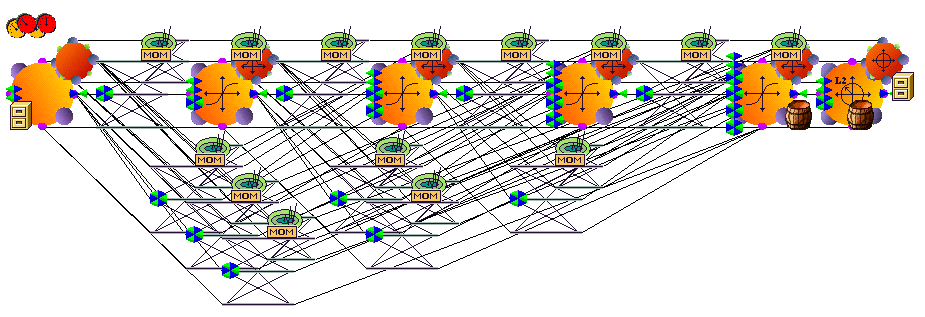Figure 2. Simulated Model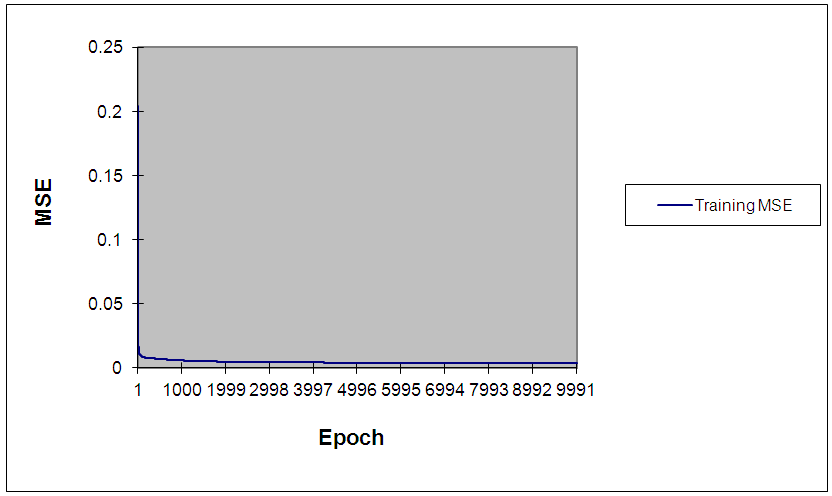Figure 3. Training results of mean square error (MSE) vs number of epochs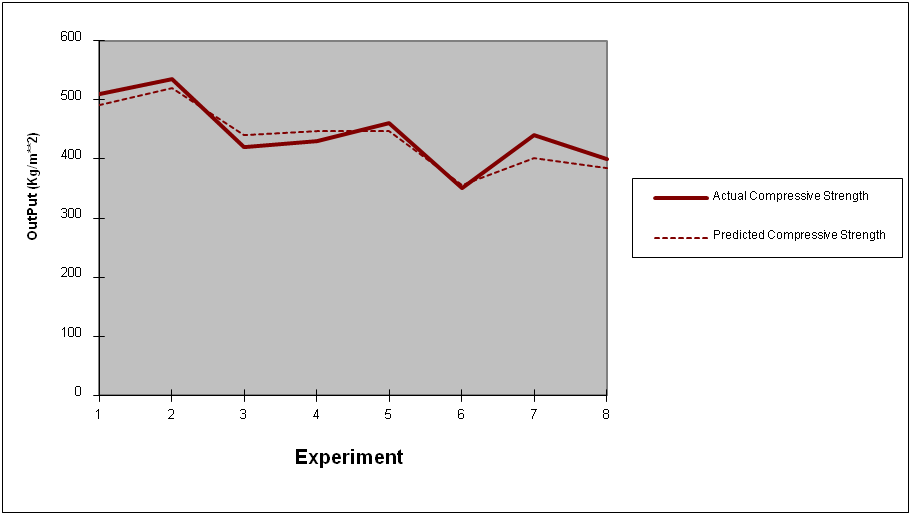Figure 4. Training data and predicted strength using FF model
 Table 1. Network training and testing results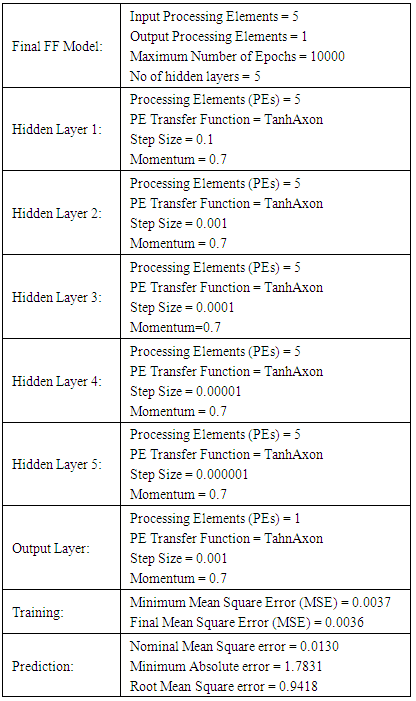### 4. Conclusions, Limitations, and Recommendations

The following basic conclusion can be drawn from this work:
Neural network computational models can be used to predict the 28-day compressive strength of concrete using mix design parameters.
The major limitations of this model are:
1) Greater computational burden.
2) Cannot interpret the relationship between input and output; and
3) Unable to deal with uncertainties.
This computational tool can be useful to structural engineers, ready-mix operators, concrete mixture designers in civil engineering, and batching plants.

### 5. Future Work

One future endeavor would be to develop a neural network model to incorporate the nonlinear relationship between the input parameters and thermal properties of concrete. These may be conductivity, diffusivity, coefficient of thermal expansion and heat of hydration of the concrete.

### References

  Snell, L.M., & Roekel, J.V., Wallace N.D., (1989), "Predicting Early Concrete Strength", Concrete International, Vol. 11(12), pp 43-47.  Wang, J.Z., Ni, H.G. & He, J.Y., (1999). "The Application of Automatic Acquisition of Knowledge to Mix Design of Concrete", Cement and Concrete Research, Vol. 29(12), pp 1875-1880.  Oh, J.W., Lee, I.W., Kim, J.T. & Lee, G.W., (1999), "Application of Neural Networks for Proportioning of Concrete Mixes", ACI Materials Journal, Vol. 96(1), pp 61-67.  Lai, S., & Serra, M., (1997), "Concrete Strength Prediction by Means of Neural Networks", Construction and Building Materials, Vol. 11(2), pp 93-98.  Brown, D.A., Murthy, P.L.N., (1991), & Berke, L., "Micro computing", Civil Engineering, Vol 6, pp 87-97.  Ghaboussi, J., Garett, J.H., & Wu, X., (1991), "Concrete Behavior and Back Propagation Neural Network Model", Journal of Engineering Mechanics, pp 117-129.  Vargas, S.V., (2002), "Analytical and Experimental Analysis of a Post-Tensioned Flat Plate Slab", Master’s report, Department of Civil Engineering, Texas A & M University-Kingsville.